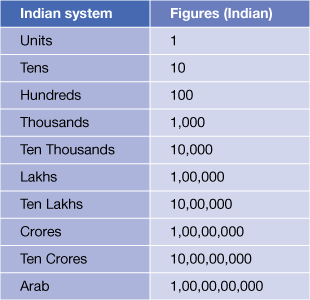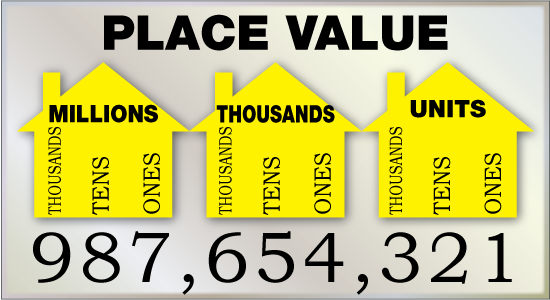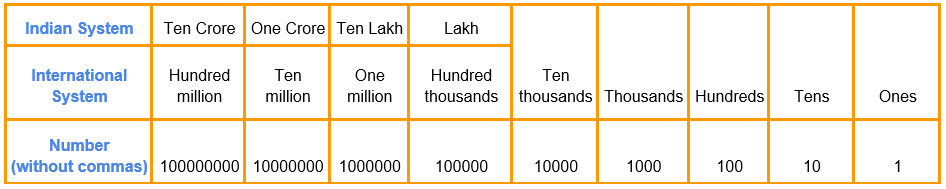# Numbers up to 6-Digits

Go back to  'Numbers and Place values'

## Introduction to Numbers up to $$6$$-Digits

By now we have travelled a standard journey, starting from $$2$$-digit numbers with place value up to Ten’s, and coming up to $$5$$-digit numbers with place value $$10,000.$$ In this journey, the place value has started at Ten's, and moved up to Hundred's, Thousand's and finally Ten Thousand's. The next level is the $$6$$-digit number, in which the highest place value is $$1,00,000$$ which has a unique name in the Indian number system – a lakh. A lakh is $$1$$ followed by $$5$$ zeros and is the smallest possible $$6$$-digit number.

## The Big Idea: Numbers up to $$6$$-Digits

### A simple idea: The Indian Numbering System

It is essential to understand the Indian numbering system at this stage because from the next higher place value (or next higher power of ten), the international numbering system would also be used, so the difference must be made clear.

There are three special numbers in the Indian numbering system – lakh, crore, and arab. A lakh is $$1,00,000,$$ a crore is equal to a hundred lakhs and is expressed as $$1,00,00,000.$$ An arab is $$100$$ crores and is expressed as $$1,00,00,00,000.$$When we move to a greater number of digits in the following modules, we will also touch upon the international numbering system. For now, it will suffice to understand that there is no particular name for a lakh in the international system, and it is simply referred to as a hundred thousand.

### Commas In $$6$$-digit numbers

Based on the details given above, you can understand that as per the Indian numbering system, there would be two commas used in any six digit number. The first would demarcate the first three digits (from right) to show how many thousands there are, and the next would come after the first $$5$$ digits from the right to show how many lakhs there are in the number. For example, you would write $$372672$$ as $$3,72,672$$ to mean that the number has $$3$$ lakhs, and it has $$72$$ thousand.

### How To Decompose $$6$$-digit numbers

Like we said at the start, a six digit number has place values up to a lakh. These are the names of the place values (starting from the right) in a six digit number:

• Digit $$1$$-Units
• Digit $$2$$-Tens
• Digit $$3$$-Hundreds
• Digit $$4$$-Thousands
• Digit $$5$$-Ten Thousands
• Digit $$6$$-Lakhs

So let us take a random six digit number like $$528497,$$ and see how it gets decomposed.

\begin{align} &{ \text { Digit } 1 \text { Place Value} = 7 \times 1 = \qquad \qquad \quad 7} \\ &{ \text { Digit } 2 \text { Place Value} = 9 \times 10 = \qquad \qquad 90} \\ &{ \text { Digit } 3 \text { Place Value} = 4 \times 100 = \qquad \quad 400} \\& { \text { Digit } 4 \text { Place Value} = 8 \times 1000 = \quad \quad8000 } \\ &{ \text { Digit } 5 \text { Place Value} = 2 \times 10000 = \quad20000 } \\ &{ \text { Digit } 6 \text { Place Value} = 5 \times 100000 = 500000 } \end{align}

## How is it important

### Zeroes in $$6$$-digit numbers

This has been discussed before for $$2$$ to $$5$$ digit numbers, and the same rules apply to six digit numbers as well. Any zero which does not have any non-zero number to its right does not count in the digits of the $$6$$ digit number. Take for example two $$6$$ digit numbers $$023843,$$ and $$002305.$$In both these numbers, the $$6^{\text{th}}$$ number on the extreme left does not have any further non zero number to its left. Additionally, in $$002305,$$ the $$5^{\text{th}}$$ number from the right also does not have any further non zero number to its left. So for $$002305,$$ both the two numbers on the left extreme are without any value. So the two numbers can actually be written as $$23843$$ (which makes it a $$5$$ digit number) and $$2305$$ (which makes it a $$4$$ digit number).

### A Very Interesting $$6$$-Digit Number

As you continue to explore your way around the exciting world of numbers, you will come across a number which usually is written using $$6$$ digits (although shorter versions are also used). This number is called $$Pi$$ and is represented as $$3.14159$$ and the Greek letter $$\pi.$$ It is defined as the ratio between a circle’s circumference and its diameter. So, if the diameter is $$D,$$ and circumference is $$C,$$ then $$C = πD.$$ In more simple terms, it is the ratio of the number $$22$$ and the number $$7.$$ Although it is usually represented by six digits (and sometimes even smaller versions like $$3.14$$ or $$3.142$$), it is theoretically possible to have infinite digits in this number.
Now we come to the interesting part. Instead of
$${22 \over 7}$$, let us consider $${1 \over 7}$$. This number $${1 \over 7}$$ is another cyclic number (it has infinite numbers after the decimal point), but we usually write it as $$0.142857.$$ Here are some amazing facts about $$0.142857.$$

• When you multiply these $$6$$ digits $$142857$$ by $$7,$$ you get $$999999.$$
• It is even more interesting to note that the same set of digits get repeated when you multiply $$142857$$ by the numbers $$1$$ through $$6.$$
• \begin{align}142857 \times 2 = 285714\end{align}
• \begin{align}142857 \times 3 = 428571\end{align}
• \begin{align}142857 \times 4 = 571428\end{align}
• \begin{align}142857 \times 5 = 714285\end{align}
• \begin{align}142857 \times 6 = 857142\end{align}

## Common mistakes or misconceptions

• Misconception: One hundred thousand” and “one lakh” are two different numbers.
In India, children are taught to name numbers based on both the Indian naming system and the International naming system. So, 100,000 is written as “One hundred thousand” as per the International naming system but “One lakh” as per the Indian system.
• Children tend to make mistakes when placing commas in numbers of 6 or more digits.
This once again happens because of two conventions - the Indian system and the International system. Here children should build comfort writing the same number in both systems. Targeted practice helps.

## Tips and Tricks

Tip: Create a chart with all the place names listed. Let children see how till ten-thousand the naming convention is the same. But for 6 digits or higher the same number has two names. The choice of names does not change the value of the number. “One lakh” and “one hundred thousand” are equal (have the same value).Tip: Remember the number of zeros after 1 for two large numbers. E.g. remember that “one crore” is 1 followed by 7 zeroes. And “1 million” is 1 followed by 6 zeroes. All other larger or smaller numbers can then be written with reference to these two numbers.

Trick: Children tend to make mistakes when rounding off a number. If we want to round off 4,63,859 to the nearest thousand, it helps to simply underline all digits that are in the lower place values (ones, tens and hundreds, in this example). Then simply replace those digits with 0. While doing this check the highest place value we are replacing - hundreds place in this case. If that is 5 or higher increase the thousands place by one. So the rounded off number will become 4,64,000.